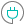#NORMINV (PQL - xl)

This function returns the inverse of the normal cumulative distribution for the given mean and standard deviation

#### Syntax

NORMINV(probability, mean, standard dev)

##### Function Arguments
 Name Description Type Optional probability Probability of the normal distribution Number mean Arithmetic mean of the distribution Number standard dev Standard deviation of the distribution Must be greater than zero Number